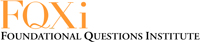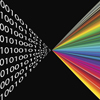# Information is bits

Marianne Freiberger

## Information is bits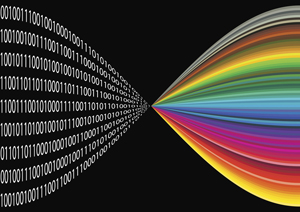Information is bits.

We send information over the internet every day. All that information, whether it's a birthday email or your homework, is in encoded into sequences of 0s and 1s. What's the best way of doing this? The way that uses the least amount of 0s and 1s and therefore requires the smallest amount of computer memory.

Let’s do a back-of-the-envelope calculation. Suppose we want to encode the 26 (lower case) letters of the alphabet, plus an additional symbol that denotes a space: each of the 27 symbols is to be represented by a string of 0s and 1s. How long do these strings need to be? Clearly they must be long enough to give a total of at least 27 distinct strings, so that we can associate them uniquely to our 27 symbols. There are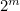strings of length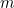(because we have two choices for each entry in the string) so we need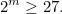Solving forgives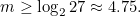This suggests that we need 5 bits per character to encode each of our 27 symbols. You can make a similar calculation for the 45,000 characters of the Mandarin language, giving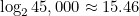and suggesting that you need 16 bits per character to encode them. We still don’t know the exact code to use, but at least we know how heavy it’s going to be on our computer memory.

### Exploiting patterns

The calculation above is neat, but we can do better. "Any reasonable [code] would take advantage of the fact that some letters, like the letter "e" in English, occur much more frequently than others," explains Scott Aaronson, a computer scientist at the Massachusetts Institute of Technology. "So we can use a smaller number of bits for those." It's an idea that's been used in Morse code for over 150 years: here the more common letters are encoded using shorter strings of dots and dashes than the rarer ones..

As an example, suppose there are only three letters to encode, A, B and C. Suppose that A has frequency 1/2 (in a long text half of the letters will be A) and B and C have frequency 1/4 each (in a long text a quarter of the letters will be B and another quarter will be C). Since A is the most frequent, let’s use a single bit, say 0, to encode it. For B and C we will use two bits each, say 01 and 11. You can check for yourself that this is a decent code — the receiver can decode it to find the original letter sequence without any ambiguity. To encode a text made up of those letters, we need one bit per symbol half of the time (for the A), two bits per symbol a quarter of the time (for the B) and two bits per symbol another quarter of the time (for the C). So the average number of bits per symbols is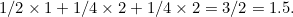We can even guess at a more general formula that works for different frequency distributions. To see how, let’s first stick to our example of A, B and C, and imagine that half of the time the letter A occurs it is red and that the other half it is green. This gives four possibilities: a red letter A with frequency 1/4, a green letter A with frequency 1/4, an ordinary black letter B with frequency 1/4, and a black letter C with frequency 1/4. All the frequencies are now the same, so if we wanted to encode the characters in a way that distinguishes the colour of the A’s we would need around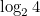bits per character. That’s the same calculation as the one above. In particular, we need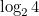bits to identify the B and the C.

For the letter A, the numberis overkill: we don’t actually need to distinguish between the two colours. So let’s deduct the number of bits needed to distinguish between two different characters (corresponding to the two different colours), which is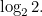This gives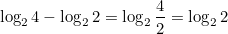bits to represent the A.

The average number of bits per symbol is now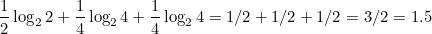That’s the same number we got before, which is reassuring. But we also notice that each term in the sum above is the frequency of one of the characters (e.g. 1/2 for A) times the logarithm of 1 divided by the frequency (e.g.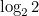for A). And it turns out that exactly the same way of thinking works in general for an alphabet of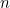symbols. Writing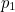for the frequency of symbol 1,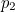for the frequency of symbol 2, and so on, an estimate for the average number of bits needed per symbol is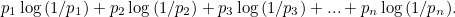### The birth of the bit

Thinking of information in terms of bits — 0s and 1s — seems like something that firmly belongs to the modern era, but the idea has been around for over 60 years. It was the mathematician Claude Shannon who in 1948 popularised the word "bit", which is shorthand for "binary digit". Shannon also came up with the formula we found above. Given an alphabet ofsymbols and a probability distribution telling you the probability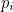with which a symboloccurs in a text made out of those symbols, the number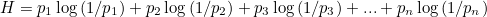Claude Shannon.

is called the entropy of the distribution (see this article to find out more). Shannon proved that the average number of bits needed per symbol cannot be smaller than the entropy, no matter how cleverly you encode them. For the vast majority of alphabets and distributions, the average number of bits needed is in fact only slightly bigger than the entropy. (One encoding that comes very close to Shannon's entropy for the vast majority of alphabets and distributions is the so-called Huffman code.)

If you’re in the business of sending messages long-distance, the entropy is a useful number to know. If you know you can transmit some number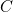bits per second, and that the symbols in your message require around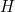bits per symbol on average, then you’d guess that you can transmit around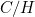symbols per second on average. Shannon showed that this is indeed correct: by picking a clever way of encoding your symbols you can guarantee an average transmission rate that’s as close toper second as you like. Conversely, no matter how you encode your symbols, it’s impossible to transmit at a faster rate than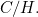This neat fact is knows as Shannon’s source coding theorem.

Shannon published this result in A mathematical theory of communication, "A paper that is justly celebrated as one of the greatest of the twentieth century," says Aaronson. It's a highly theoretical effort. The source code theorem, for example, doesn't tell you which code gets you within a specified distance of the maximal rate of C/H per second — it only says that there is one.

When it comes to practical applications, the theorem also has a major short-coming: it assumes that your communication channels transmit pristine messages and never introduce any errors. In real life this just doesn't happen: whether you send a message in a telegram, via email or using SMS, there is always a chance it is scrambled on the way and arrives at the other end corrupted. This is where things get interesting. How do you deal with so-called noisy channels and how much information can you transmit? Find out in the next article.